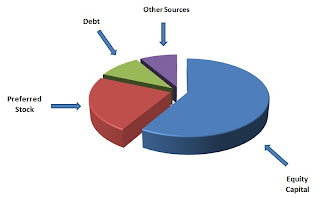# Capital Structure

A company can obtain long-term financing in the form of equity, debt or a combination of both. Capital Structure is the proportion of debt and preference and equity shares on a firm’s balance sheet.

Optimal Capital Structure is the capital is the capital structure at which the weighted average cost of capital is minimum and thereby maximum value of the firm.

## Understanding Capital Structure

The relative proportions of debt, equity and other securities that a firm has outstanding constitute its capital structure. When corporations raise funds from outside investors, they must choose which type of security issue. The most common choices are financing through equity alone and financing through a combination of debt and equity.
So V = B + S = D + EShareholders are interested in maximizing the value of the firm’s shares. A key question is: What is the ratio of debt to equity, if any, that maximizes the shareholder’s value?
As it turns out, changes in capital structure benefit shareholders if and only if the value of the firm increases. So we concern ourselves with the question of which proportion of debt versus equity maximizes the overall value of the firm.
We assume perfect capital markets in the following analysis:
• Perfect competition
• No transaction costs
• No taxes (later we drop this assumption)

We also assume that the individual can borrow at the same rate as the firm.

Example:
We will compare a firm whose capital structure is all equity, with a firm that is identical, except that it has some debt in its capital structure.
Suppose a firm has 1000 shares, a share price of \$10, a market value of \$10,000, and operating income as described in the table below. This income is a perpetuity, and all earnings are paid out as a dividend to investors. Expected income is \$1500 and expected return is 15%.Suppose the firm borrows \$5,000 at 10% rate of interest and buys back 500 of its shares to create the following new situation: # shares 500, B = \$5000, S = \$5000, shares value: \$10,

annual interest: \$500

Argument that value has increased: If operating income is greater than \$1,000 then the expected Return On Equity increases. Since we expect operating income of \$1,500, leverage must have added value. Shareholders expect 20% ROE with leverage.

Counter-argument: Suppose an investor takes \$10 of his/her own money and borrows \$10 at 10% interest and invests the \$20 to buy 2 shares of the unlevered firm.

Note: Investor gets the same result from borrowing personally as he/she gets from investing in the leveraged firm. Therefore, the firm is not doing anything by leveraging that the investor could not do on his/her own. This implies VL = VU. This is the famous Modigliani-Miller Proposition I.
So leverage increases the expected earnings per share, but not the share price. How can that be?
Leverage also increases the riskiness of the earnings. We must discount those higher expected earnings at a higher discount rate.
All equity: 1.50/.15 = \$10
Leveraged: 2.00/.20 = \$10
Recall: The value of a firm = the PV of the future cash flows.
If the firm is all equity financed, then VU = C1/(1+r0) + C2/(1+r0)2 + … where r0 is the cost of capital of an all equity financed firm.
If there is some debt in the capital structure, the cost of capital is given by:
r = [B/(B+S)]rB + [S/(B+S)]rS where rS is the cost of equity capital of the now leveraged firm.
and VL = C1/(1+r) + C2/(1+r)2 + …
Since the value of the company doesn’t change with leverage, VL = VU, and so r must equal r0 – that is, r must be constant as debt is added to the capital structure (remember here we are assuming no taxes etc.). The idea is that as “expensive” equity is replaced with “cheap” debt, the risk to equity increases, as a result of the increased leverage, and so rS increases. The increase in rS just balances against the increased fraction of cheaper debt, so that r stays constant.
r0 = cost of capital for the all equity firm = ra = required/expected return on the assets – reflects business risk – not financial leverage. If we solve:
r0 = (B/(B+S))rB + (S/(B+S))rS for rS, we get rS = r0 +(B/S)(r0 – rB)
This is Modigliani-Miller Proposition II.
In our example above, ra = 1500/10,000 = 15%. If B = S = 5000, then:
rS = 15% +(5000/5000)(15% – 10%) = 20%
Initially the firm was all equity, had 1,000 shares at \$10/share and equity worth \$10,000
V = 1500/.15 = (CF/rS) = \$10,000
With \$5,000 debt at 10% and \$5,000 equity, now rS = 20%, up from 15% and:
V = 500/0.10 + 1000/0.20 = interest payment/rB + CF/rS = 5,000 + 5,000 = 10,000
Essentially, we split a single cash flow of 1500/year, discounted at 15% into 2 cash flows of 500 and 1000, the former discounted at 10% and the latter discounted at 20%.

At HelpWithAssignment.com we provide best quality Assignment help, Homework help, Online Tutoring and Thesis and Dissertation help as well. For any of the above services you can contact us at http://www.helpwithassignment.com/ and
http://www.helpwithassignment.com/finance-assignment-help
This article is in continuation with our previous articles on Finance which include Finance- Present Value, Finance- Net Present Value, Finance – Internal Rate of Return, Finance -Present Value Annuity